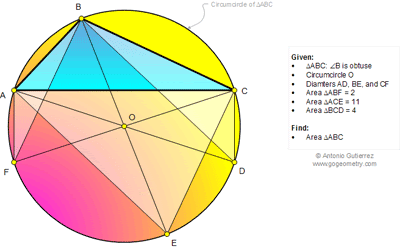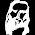## Thursday, July 24, 2014

### Geometry Problem 1033: Triangle, Angle Obtuse, Circumcircle, Diameter, Area

Level: Mathematics Education, High School, Honors Geometry, College.

Click the figure below to see the complete problem 1033.1.Let S(ABC) be the area of ABC.

S(CDE) = S(ABF) = 2
S(ACDE) = 11+2 = 13
S(OCDE) = 13/2
S(OCE) = 13/2 - 2 = 9/2
S(BCE) = 9

S(AEF) = S(BCD) = 4
S(ACEF) = 11+4 = 15
S(AOEF) = 15/2
S(AOE) = 15/2 - 4 = 7/2
S(ABE) = 7

S(ABC) = 9+7-11 = 5

2.AC=FD, CE=BF, and AE=BD, so [BFD]=[ECA]=11.
[ABC]=2+4+11-[ACDF]=2+4+11-2[ACF]=2+4+11-2(2+4)=5